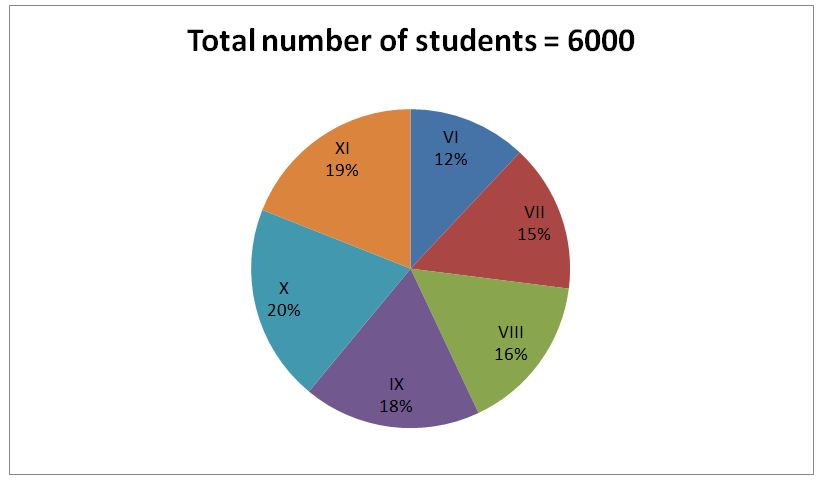# IBPS RRB Clerk Prelims Quantitative Aptitude Questions 2019 (Day-01)

Dear Aspirants, Our IBPS Guide team is providing new series of Quantitative Aptitude Questions for IBPS RRB Clerk Prelims 2019 so the aspirants can practice it on a daily basis. These questions are framed by our skilled experts after understanding your needs thoroughly. Aspirants can practice these new series questions daily to familiarize with the exact exam pattern and make your preparation effective.

##### IBPS RRB Clerk Prelims Free Mock Test

[WpProQuiz 6486]

Directions (Q. 1 – 5): In the following questions, two equations I and II are given. You have to solve both the equations and give answer as,

a) If x > y

b) If x ≥ y

c) If x < y

d) If x ≤ y

e) If x = y or the relation cannot be established

1)

I) 5x2 – 18x – 35 = 0

II) 4y2 – 12y – 72 = 0

2)

I) 3x2 – 5x – 12 = 0

II)4y2 – 12y + 9 = 0

3)

I) 7x + 6y + 29 = 2x + 3y

II)3x – 2y + 6 = 0

4)

I) x2 – 12y + 35 = 0

II) y2 – 13y + 42 = 0

5)

I) 2x2 – 11x + 15 = 0

II) 3y2 – 12y + 12 = 0

Directions (Q. 6 – 10): Study the following information carefully and answer the given questions.

The following pie chart shows the total number of students studied in various standards.6) Find the difference between the total number of students studied in standard VI and standard IX?

a) 360

b) 420

c) 480

d) 540

e) None of these

7) Find the average number of students studied in standard VII, VIII and X together?

a) 1150

b) 1280

c) 1330

d) 1020

e) None of these

8) Find the ratio between the total number of girls studied in standard VI to that of total number boys studied in standard XI, if the ratio between the total girls to that of boys studied in standard VI is 4 : 5 and the percentage of total girls studied in standard XI is 45 %?

a) 150 : 439

b) 320 : 627

c) 270 : 553

d) 90 : 216

e) None of these

9) Total number of students studied in standard VII is what percentage of total number of students studied in standard X?

a) 60 %

b) 120 %

c) 75 %

d) 90 %

e) None of these

10) Find the difference between the average numbers of students studied in standard VI, VIII together to that of the average number of students studied in standard VII, XI together?

a) 360

b) 280

c) 140

d) 180

e) None of these

Directions (1-5):

I) 5x2 – 18x – 35 = 0

5x2 – 25x + 7x – 35 = 0

5x (x – 5) + 7 (x – 5) = 0

(5x + 7) (x – 5) = 0

x = -7/5, 5 = -1.4, 5

II) 4y2 – 12y – 72 = 0

4y2 – 24y + 12y – 72 = 0

4y (y – 6) + 12 (y – 6) = 0

(4y + 12) (y – 6) = 0

y = -3, 6

Can’t be determined

I) 3x2 – 5x – 12 = 0

3x2 – 9x + 4x – 12 = 0

3x (x – 3) + 4 (x – 3) = 0

(3x + 4) (x – 3) = 0

x = -4/3, 3 = -1.33, 3

II) 4y2 – 12y + 9 = 0

4y2 – 6y – 6y + 9 = 0

2y (2y – 3) – 3 (2y – 3) = 0

(2y – 3) (2y – 3) = 0

y = 3/2, 3/2 = 1.5, 1.5

Can’t be determined

I) 7x + 6y + 29 = 2x + 3y

5x + 3y = -29 —> (1)

II) 3x – 2y + 6 = 0

3x – 2y = – 6 —> (2)

By solving the equation (1) and (2), we get,

x = -4, y = -3

x < y

I) x2 – 12y + 35 = 0

(x – 5) (x – 7) = 0

x = 5, 7

II) y2 – 13y + 42 = 0

(y – 6) (y – 7) = 0

y = 6, 7

Can’t be determined

I) 2x2 – 11x + 15 = 0

2x2 – 6x – 5x + 15 = 0

2x (x – 3) – 5 (x – 3) = 0

(2x – 5) (x – 3) = 0

x = 5/2, 3 = 2.5, 3

II) 3y2 – 12y + 12 = 0

3y2 – 6y – 6y + 12 = 0

3y (y – 2) – 6 (y – 2) = 0

(3y – 6) (y – 2) = 0

y = 2, 2

x > y

Directions (6-10):

Required difference = [(18 – 12)/100]*6000 = 360

The average number of students studied in standard VII, VIII and X together

= >[(15 + 16 + 20)/100]*(1/3)*6000

= > 1020

The total number of girls studied in standard VI

= >6000*(12/100)*(4/9) = 320

The total number boys studied in standard XI

= >6000*(19/100)*(55/100) = 627

Required ratio = 320 : 627

Required % = (15/20)*100 = 75 %

The average number of students studied in standard VI, VIII together

= >6000*(28/100) * (1/2)

The average number of students studied in standard VII, XI together

= >6000*(34/100)* (1/2)

Required difference = 3000*[(34 – 28)/100] = 180